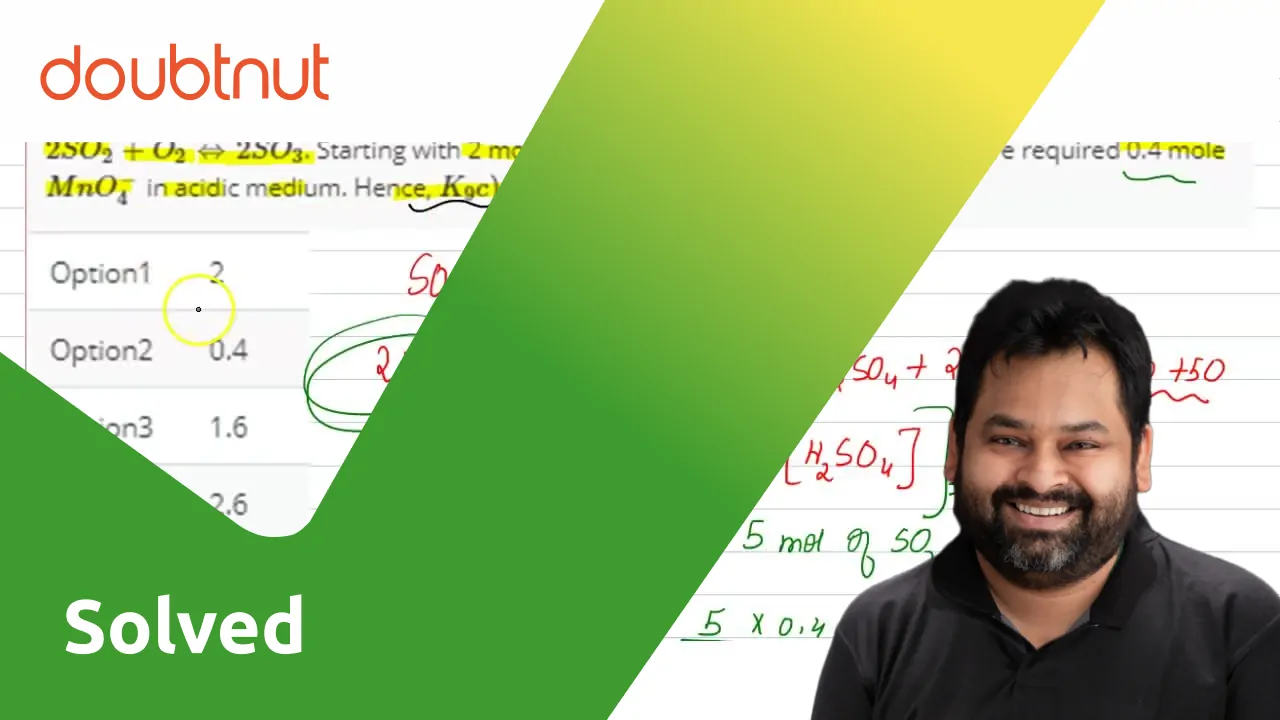Doubtnut App - Get 50 Lakh+ video solutions better than GoogleInstall Now

# 2SO2+O2⇔2SO3. Starting with 2 moles SO2 and 1 mole O2 in 1L flask, mixture required 0.4 mole MnO−4 in acidic medium. Hence, K9c) is

A

2

B

0.4

C

1.6

D

2.6

Text Solution
Verified by Experts

## SO2 will react with MnO−4 2KMnO4+3H2SO4→K2SO4+2MnSO4+3H2O+5O SO2+H2O+O→[H2SO4]×5 2 mol of KMnO4=5 mol of SO2 0.4 mol of KMnO4=52×0.4 mol of SO2 =1 mol of SO2 ∴ Reaction mixture at equilibrium contains 1 mol of SO2 2SO2+O2⇐⇒2SO3Initial21−At eqm.11−121 ∴At eqm. [SO2]=1,[O2]=12,[SO3]=1 Kc=[SO3]2[SO2]2[O2]=(1)2(1)212=2